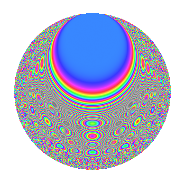# Properties

 Label 8012.2.aLevel 8012 Weight 2 Character orbit a Rep. character $$\chi_{8012}(1,\cdot)$$ Character field $$\Q$$ Dimension 167 Newform subspaces 2 Sturm bound 2004 Trace bound 1

# Related objects

## Defining parameters

 Level: $$N$$ $$=$$ $$8012 = 2^{2} \cdot 2003$$ Weight: $$k$$ $$=$$ $$2$$ Character orbit: $$[\chi]$$ $$=$$ 8012.a (trivial) Character field: $$\Q$$ Newform subspaces: $$2$$ Sturm bound: $$2004$$ Trace bound: $$1$$

## Dimensions

The following table gives the dimensions of various subspaces of $$M_{2}(\Gamma_0(8012))$$.

Total New Old
Modular forms 1005 167 838
Cusp forms 1000 167 833
Eisenstein series 5 0 5

The following table gives the dimensions of the cuspidal new subspaces with specified eigenvalues for the Atkin-Lehner operators and the Fricke involution.

$$2$$$$2003$$FrickeDim.
$$-$$$$+$$$$-$$$$88$$
$$-$$$$-$$$$+$$$$79$$
Plus space$$+$$$$79$$
Minus space$$-$$$$88$$

## Trace form

 $$167q + 4q^{7} + 171q^{9} + O(q^{10})$$ $$167q + 4q^{7} + 171q^{9} + 2q^{11} - 6q^{13} + 2q^{15} + 2q^{17} + 14q^{21} - 16q^{23} + 165q^{25} + 12q^{27} + 4q^{29} - 8q^{31} + 6q^{33} - 8q^{35} + 2q^{39} + 2q^{41} - 6q^{43} + 24q^{45} + 10q^{47} + 183q^{49} + 14q^{51} - 2q^{53} + 4q^{55} + 28q^{57} + 10q^{59} + 12q^{63} + 18q^{65} + 8q^{67} + 30q^{69} - 4q^{71} + 16q^{73} - 16q^{75} + 8q^{77} + 12q^{79} + 175q^{81} - 6q^{83} + 14q^{85} + 6q^{87} - 36q^{89} - 10q^{91} - 14q^{93} - 10q^{95} + 24q^{97} - 6q^{99} + O(q^{100})$$

## Decomposition of $$S_{2}^{\mathrm{new}}(\Gamma_0(8012))$$ into newform subspaces

Label Dim. $$A$$ Field CM Traces A-L signs $q$-expansion
$$a_2$$ $$a_3$$ $$a_5$$ $$a_7$$ 2 2003
8012.2.a.a $$79$$ $$63.976$$ None $$0$$ $$-19$$ $$0$$ $$-40$$ $$-$$ $$-$$
8012.2.a.b $$88$$ $$63.976$$ None $$0$$ $$19$$ $$0$$ $$44$$ $$-$$ $$+$$

## Decomposition of $$S_{2}^{\mathrm{old}}(\Gamma_0(8012))$$ into lower level spaces

$$S_{2}^{\mathrm{old}}(\Gamma_0(8012)) \cong$$ $$S_{2}^{\mathrm{new}}(\Gamma_0(2003))$$$$^{\oplus 3}$$$$\oplus$$$$S_{2}^{\mathrm{new}}(\Gamma_0(4006))$$$$^{\oplus 2}$$

## Hecke characteristic polynomials

There are no characteristic polynomials of Hecke operators in the database